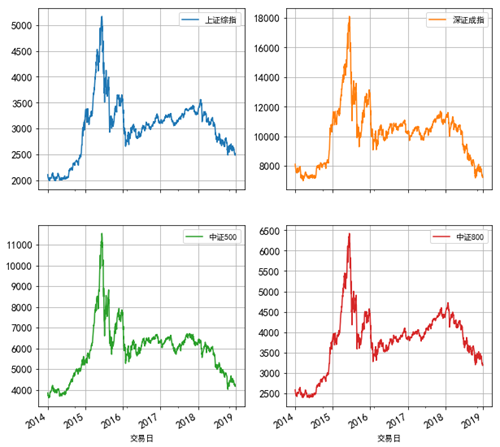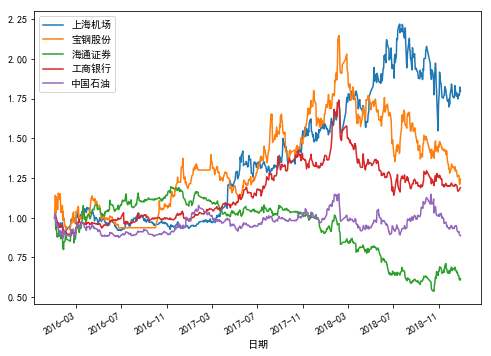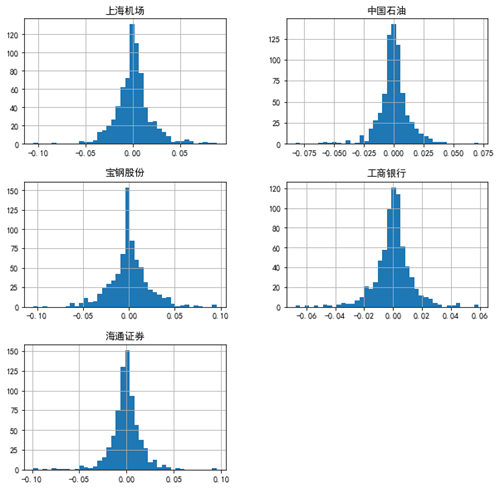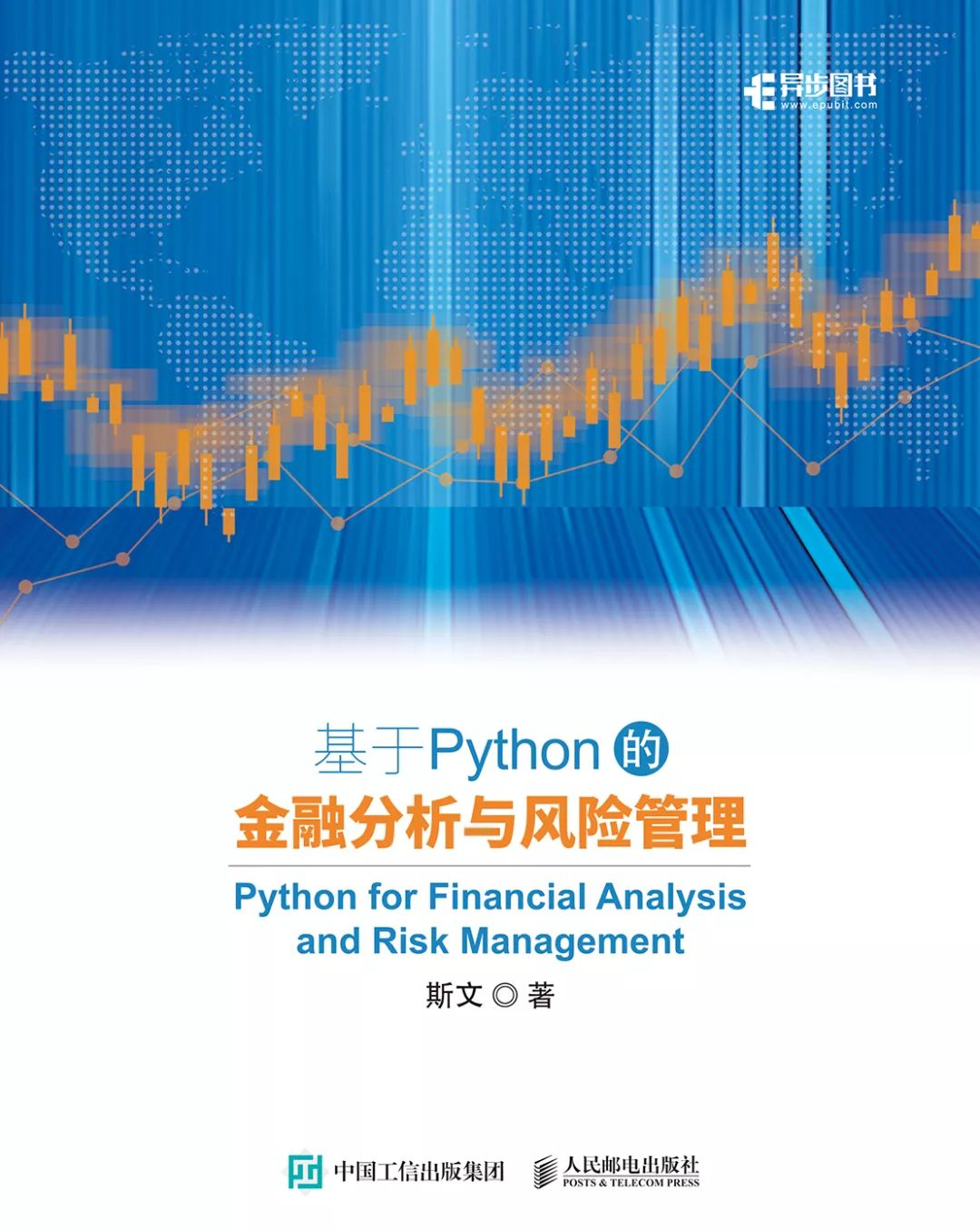# 金融行业掀起学Python热潮，看看政策怎么说！In : import numpy as np
...: import pandas as pd
...: import matplotlib.pyplot as plt
...: from pylab import mpl
...: mpl.rcParams['font.sans-serif'] = ['SimHei']
...: mpl.rcParams['axes.unicode_minus'] = False

In : index_data.plot(subplots=True,layout=(2,2),figsize=(10,10),fontsize=13,grid=True)
Out:▲运用Python绘制A股相关指数日收盘价走势图（2014年至2018年）

【例】假定投资组合配置了5只A股股票（具体信息见下表），数据是2016年至2018年期间的每个交易日收盘价格。下面就通过Python计算投资组合的预期收益率和年化波动率，具体分为4个步骤。

 股票代码 600009 600019 600837 601398 601857 证券简称 上海机场 宝钢股份 海通证券 工商银行 中国石油▲股价2016至2018年的走势图（股价在2016年首个交易日归一处理）

In : data=pd.read_excel('C:/Desktop/构建投资组合的5只股票数据（2016-2018）.xlsx', sheet_name=

Out:
上海机场   宝钢股份    海通证券   工商银行   中国石油

2016-01-04  28.27     5.23      14.28     4.45      8.13
2016-01-05  28.76     5.43      14.33     4.47      8.07
2016-01-06  29.50     5.97      14.58     4.51      8.31
2016-01-07  27.99     5.38      13.23     4.43      7.83
2016-01-08  28.03     5.92      13.51     4.46      7.99

In : (data/data.iloc).plot(figsize=(8,6))  #将股价按首个交易日进行归一处理并可视化▲5只股票日收益率的直方图

In : R=np.log(data/data.shift(1))      #按照对数收益率的计算公式得到股票收益率
...: R=R.dropna()                 #删除缺省的数据
...: R.describe()
Out:
上海机场    宝钢股份    海通证券    工商银行    中国石油
count  730.000000  730.000000  730.000000  730.000000  730.000000
mean     0.000802    0.000298   -0.000663    0.000237   -0.000165
std      0.019078    0.021775    0.016945    0.012614    0.012901
min     -0.105361   -0.104059   -0.098549   -0.067593   -0.082521
25%     -0.008854   -0.009260   -0.007118   -0.004662   -0.005274
50%      0.000372    0.000000   -0.000648    0.000000    0.000000
75%      0.008488    0.010305    0.006625    0.006316    0.005203
max      0.088763    0.095648    0.095198    0.059223    0.070761

In : R.hist(bins=40,figsize=(10,10))    #将股票收益率按照直方图方式展示
Out:


In : R_mean=R.mean()*252            #计算股票的年化平均收益率
...: print(R_mean)

dtype: float64

In : R_cov=R.cov()*252               #计算股票的协方差矩阵并且年化处理
...: print(R_cov)
上海机场  宝钢股份 海通证券  工商银行  中国石油

In : R_corr=R.corr()                  #计算股票的相关系数矩阵
...: print(R_corr)
上海机场  宝钢股份  海通证券 工商银行  中国石油

In : R_vol=R.std()*np.sqrt(252)        #计算股票收益率的年化波动率
...: print(R_vol)

dtype: float64


从以上的相关系数矩阵分析可以看到，这5只股票的收益率之间相关性比较低，因此对于整个组合的分散化效果会比较好。

In : R_port=np.sum(weights*R_mean)     #计算投资组合的预期收益率
...: print('投资组合的预期收益率：',round(R_port,4))

In : vol_port=np.sqrt(np.dot(weights,np.dot(R_cov,weights.T)))  #计算投资组合收益波动率
...: print('投资组合收益波动率:',round(vol_port,4))-END-